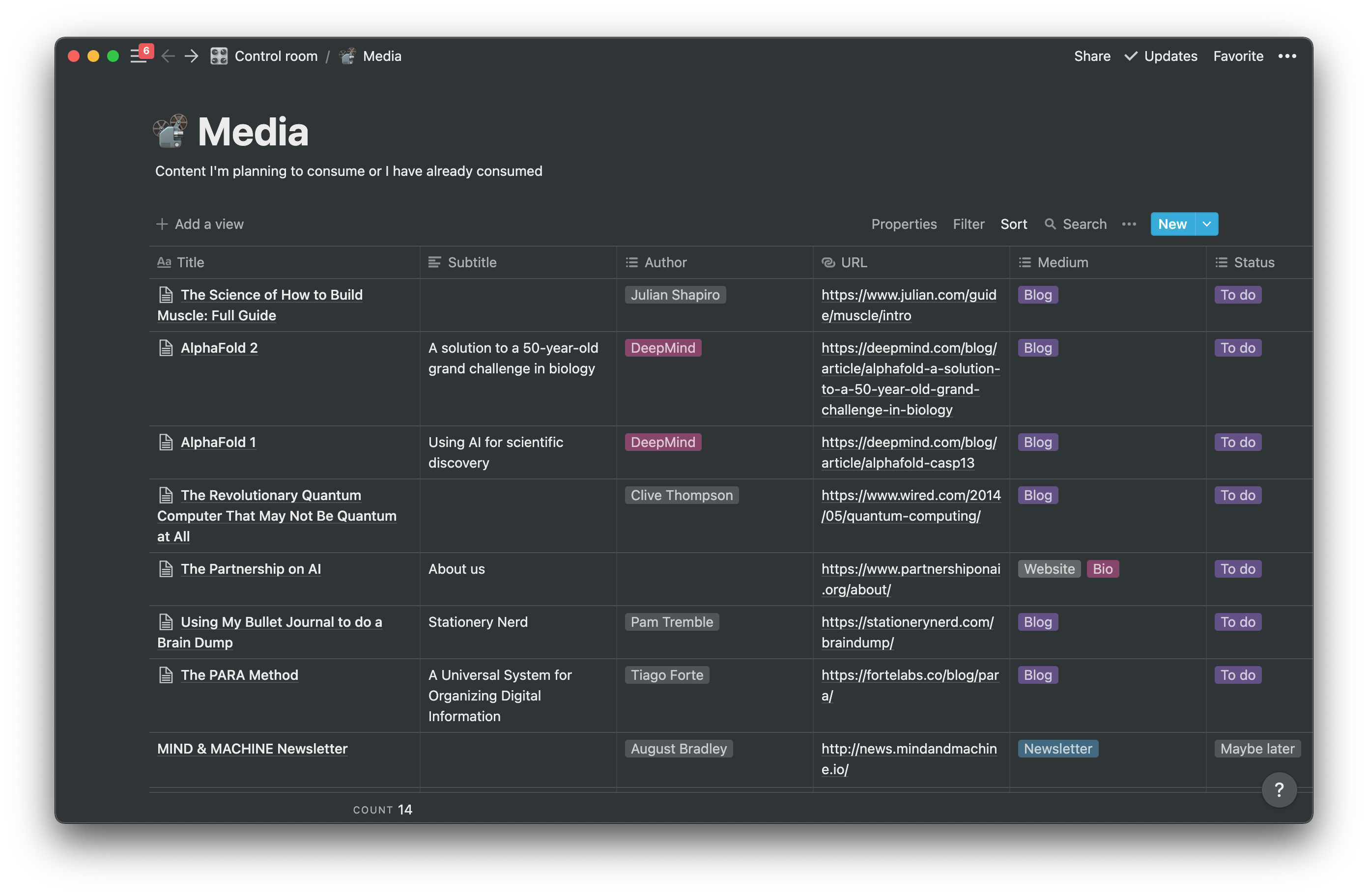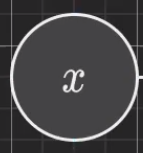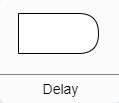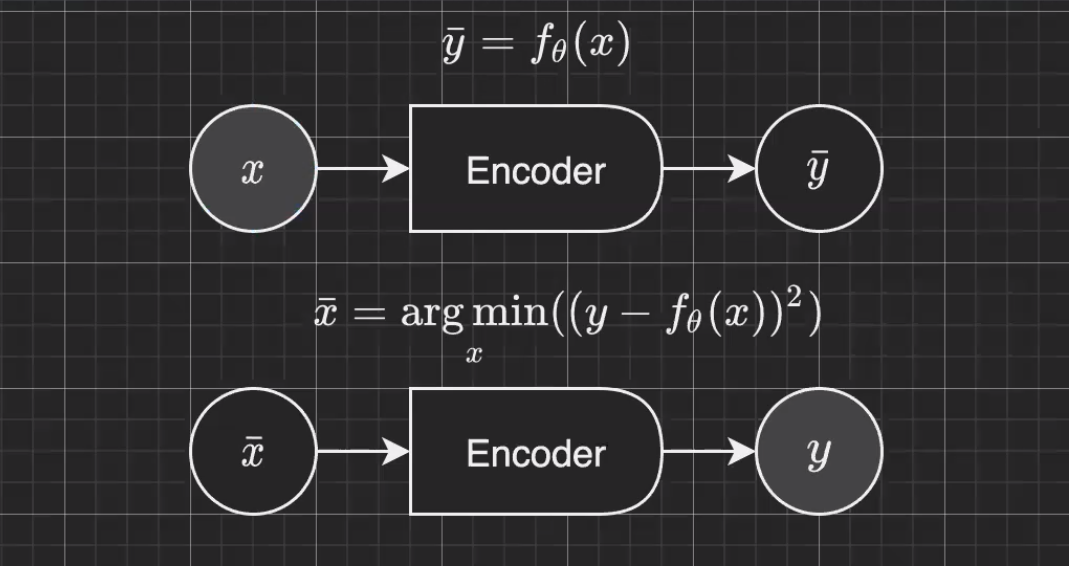# Spiral classification

🎙️ Alfredo Canziani

## Typora

Typora is a useful tool to write markdown with the addition of formulae in LaTeX. It is convenient to write paper and homework, and generating pdf file with Typora.

## NotionHere you can place all your favorite stuff. This includes but is not limited to recipes, music, books, notes. Everything in one place, simple and powerful.

When you find some useful article regarding Deep Learning, you may want to collect it for future review. The database is just all you need. You can find more information about how to use the database.

First you need to create a database by “Workspace” -> “Add a new page”. Inside this page, choose “/table” -> “Table - Full Page”. In addition to filling out the information related to the paper, we usually want to cover “The Golden Circle” aka “What? Why? How?” in our summary.

This is an example of how to organize your summary.

## Diagram.net

Diagrams.net is a great tool to draw neural network diagrams. Next we will introduce a few rules to make our diagrams more consistent with the ones in lecture.The grayscale background means this is an observation, which means they are data points from a given dataset. You can check the input and labels by going to the directory of the dataset if you want.We use “Delay” to denote the encoder(e.g., neural network).In this example, $\vx$ and $\vy$ are observations.

In the half above, we feed the $\vx$ to a given encoder to get a prediction $\bar {\vy}$. This is called forward propagation.

In the half below, we want to get the prediction $\bar{\vx}$ given observation $\vy$. We keep doing gradient descent to make the network output as close as to $\vy$. This is called amortizing inference.

Usually, we use backpropagation to compute the gradient, then we apply gradient descent with those computed values to train the model. This example shows that backpropagation is NOT only used during training. Backpropagation can also be used for inference.

## Spiral Classification

The following content is mostly the same, so here you can find what you need.

📝 Wenhao Li
6 May 2021